# DBSCAN¶

Density-based Spatial Clustering of Applications with Noise (DBSCAN) is a data clustering algorithm that finds clusters through density-based expansion of seed points. The algorithm is proposed by:

Martin Ester, Hans-peter Kriegel, Jörg S, and Xiaowei Xu A density-based algorithm for discovering clusters in large spatial databases with noise. 1996.

Density Reachability

DBSCAN’s definition of cluster is based on the concept of density reachability: a point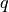is said to be directly density reachable by another point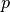if the distance between them is below a specified thresholdandis surrounded by sufficiently many points. Then,is considered to be density reachable byif there exists a sequence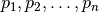such thatand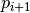is directly density reachable from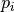.

A cluster, which is a subset of the given set of points, satisfies two properties:

1. All points within the cluster are mutually density-connected, meaning that for any two distinct pointsandin a cluster, there exists a point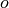sucht that bothandare density reachable from.
2. If a point is density connected to any point of a cluster, it is also part of the cluster.

Functions

There are two different implementations of DBSCAN algorithm called by dbscan function in this package:

1. Using a distance (adjacency) matrix and is O(N^2) in memory usage. Note that the boundary points are not unique.
dbscan(D, eps, minpts)

Perform DBSCAN algorithm based on a given distance matrix.

Parameters: D – The pairwise distance matrix. D[i,j] is the distance between points i and j. eps – The radius of a neighborhood. minpts – The minimum number of neighboring points (including self) to qualify a point as a density point.

The algorithm returns an instance of DbscanResult, defined as below:

type DbscanResult <: ClusteringResult
seeds::Vector{Int}          # starting points of clusters, size (k,)
assignments::Vector{Int}    # assignments, size (n,)
counts::Vector{Int}         # number of points in each cluster, size (k,)
end


2. Using an adjacency list which is build on the fly. The performance is much better both in terms of runtime and memory usage. Also, the result is given in a DbscanCluster that provides the indices of all the core points and boundary points, such that boundary points can be associated with multiple clusters.

dbscan(points, radius, leafsize=20, min_neighbors=1, min_cluster_size=1)

Perform DBSCAN algorithm based on a collection of points.

Parameters: points – matrix of points (column based) radius – The radius of a neighborhood. leafsize – number of points binned in each leaf node in the KDTree min_neighbors – minimum number of neighbors to be a core point min_cluster_size – minimum number of points to be a valid cluster

The algorithm returns an instance of DbscanCluster, defined as below:



immutable DbscanCluster <: ClusteringResult
size::Int # number of points in cluster core_indices::Vector{Int} # core points indices boundary_indices::Vector{Int} # boundary points indices

end